# Propositional and First Order Logic 1 Propositional logic

• Slides: 65Propositional and First -Order Logic 1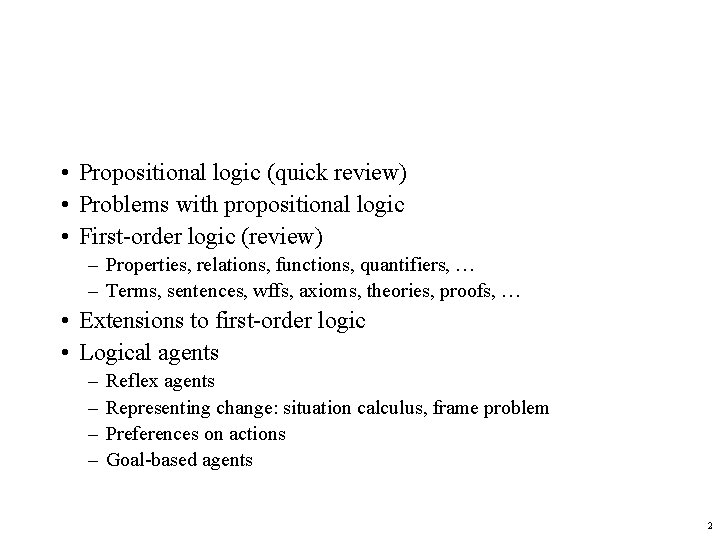• Propositional logic (quick review) • Problems with propositional logic • First-order logic (review) – Properties, relations, functions, quantifiers, … – Terms, sentences, wffs, axioms, theories, proofs, … • Extensions to first-order logic • Logical agents – – Reflex agents Representing change: situation calculus, frame problem Preferences on actions Goal-based agents 2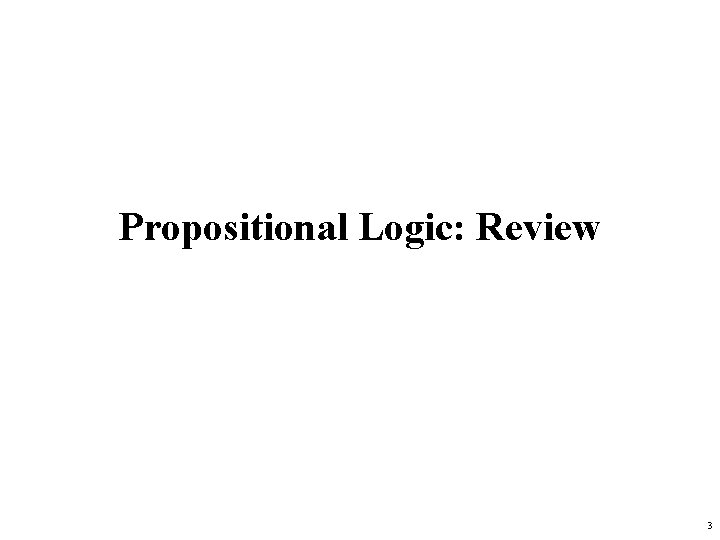Propositional Logic: Review 3Propositional logic • • Logical constants: true, false Propositional symbols: P, Q, S, . . . (atomic sentences) Wrapping parentheses: ( … ) Sentences are combined by connectives: . . . and . . . or . . . implies . . is equivalent . . . not [conjunction] [disjunction] [implication / conditional] [biconditional] [negation] • Literal: atomic sentence or negated atomic sentence 4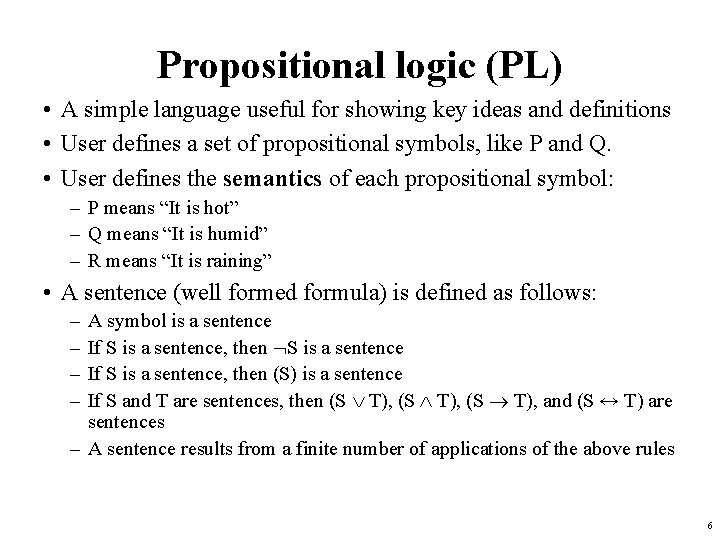Propositional logic (PL) • A simple language useful for showing key ideas and definitions • User defines a set of propositional symbols, like P and Q. • User defines the semantics of each propositional symbol: – P means “It is hot” – Q means “It is humid” – R means “It is raining” • A sentence (well formed formula) is defined as follows: – – A symbol is a sentence If S is a sentence, then S is a sentence If S is a sentence, then (S) is a sentence If S and T are sentences, then (S T), and (S ↔ T) are sentences – A sentence results from a finite number of applications of the above rules 6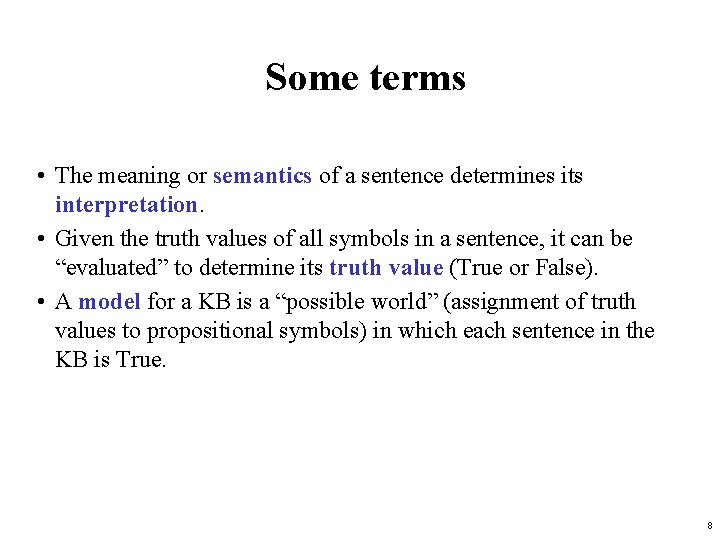Some terms • The meaning or semantics of a sentence determines its interpretation. • Given the truth values of all symbols in a sentence, it can be “evaluated” to determine its truth value (True or False). • A model for a KB is a “possible world” (assignment of truth values to propositional symbols) in which each sentence in the KB is True. 8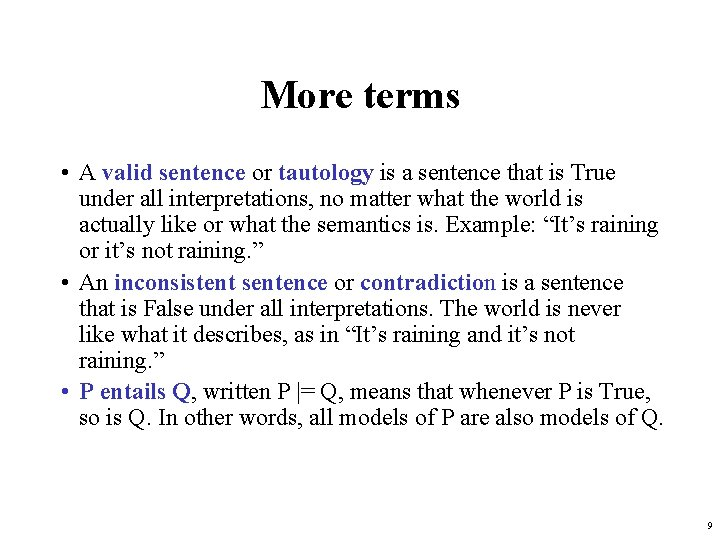More terms • A valid sentence or tautology is a sentence that is True under all interpretations, no matter what the world is actually like or what the semantics is. Example: “It’s raining or it’s not raining. ” • An inconsistent sentence or contradiction is a sentence that is False under all interpretations. The world is never like what it describes, as in “It’s raining and it’s not raining. ” • P entails Q, written P |= Q, means that whenever P is True, so is Q. In other words, all models of P are also models of Q. 9Inference rules • Logical inference is used to create new sentences that logically follow from a given set of predicate calculus sentences (KB). • An inference rule is sound if every sentence X produced by an inference rule operating on a KB logically follows from the KB. (That is, the inference rule does not create any contradictions) • An inference rule is complete if it is able to produce every expression that logically follows from (is entailed by) the KB. (Note the analogy to complete search algorithms. ) 13Sound rules of inference • Here are some examples of sound rules of inference – A rule is sound if its conclusion is true whenever the premise is true • Each can be shown to be sound using a truth table RULE PREMISE CONCLUSION Modus Ponens And Introduction And Elimination Double Negation Unit Resolution A, A B A, B A B A A B, B C B A A A A C 14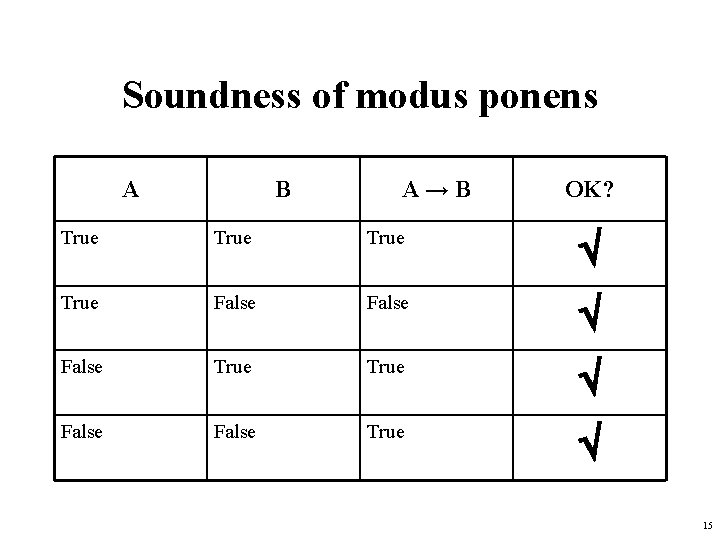Soundness of modus ponens A B A→B OK? True False True False True 15Soundness of the resolution inference rule 16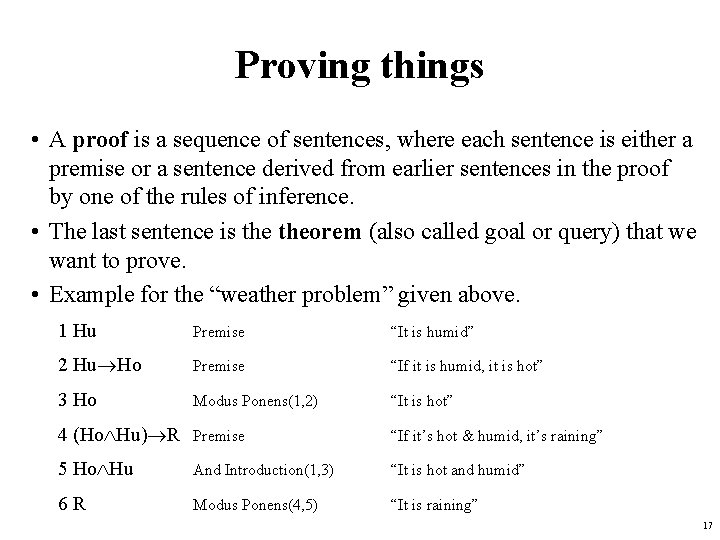Proving things • A proof is a sequence of sentences, where each sentence is either a premise or a sentence derived from earlier sentences in the proof by one of the rules of inference. • The last sentence is theorem (also called goal or query) that we want to prove. • Example for the “weather problem” given above. 1 Hu Premise “It is humid” 2 Hu Ho Premise “If it is humid, it is hot” 3 Ho Modus Ponens(1, 2) “It is hot” 4 (Ho Hu) R Premise “If it’s hot & humid, it’s raining” 5 Ho Hu And Introduction(1, 3) “It is hot and humid” 6 R Modus Ponens(4, 5) “It is raining” 17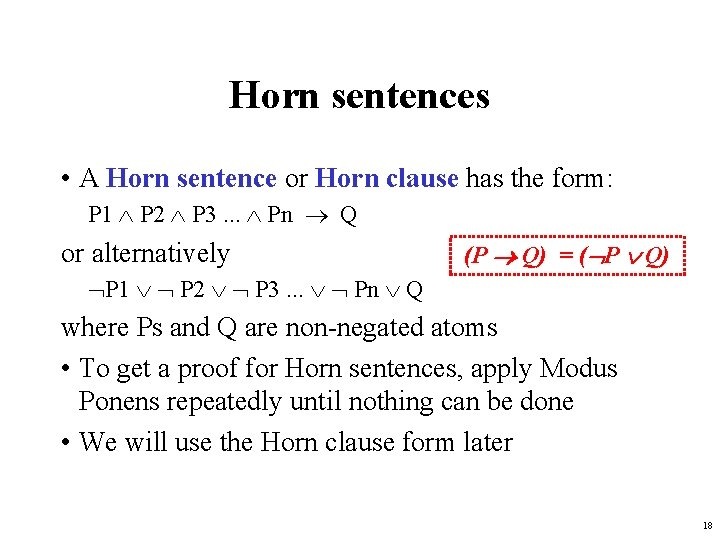Horn sentences • A Horn sentence or Horn clause has the form: P 1 P 2 P 3. . . Pn Q or alternatively (P Q) = ( P Q) P 1 P 2 P 3. . . Pn Q where Ps and Q are non-negated atoms • To get a proof for Horn sentences, apply Modus Ponens repeatedly until nothing can be done • We will use the Horn clause form later 18Entailment and derivation • Entailment: KB |= Q – Q is entailed by KB (a set of premises or assumptions) if and only if there is no logically possible world in which Q is false while all the premises in KB are true. – Or, stated positively, Q is entailed by KB if and only if the conclusion is true in every logically possible world in which all the premises in KB are true. • Derivation: KB |- Q – We can derive Q from KB if there is a proof consisting of a sequence of valid inference steps starting from the premises in KB and resulting in Q 19Two important properties for inference Soundness: If KB |- Q then KB |= Q – If Q is derived from a set of sentences KB using a given set of rules of inference, then Q is entailed by KB. – Hence, inference produces only real entailments, or any sentence that follows deductively from the premises is valid. Completeness: If KB |= Q then KB |- Q – If Q is entailed by a set of sentences KB, then Q can be derived from KB using the rules of inference. – Hence, inference produces all entailments, or all valid sentences can be proved from the premises. 20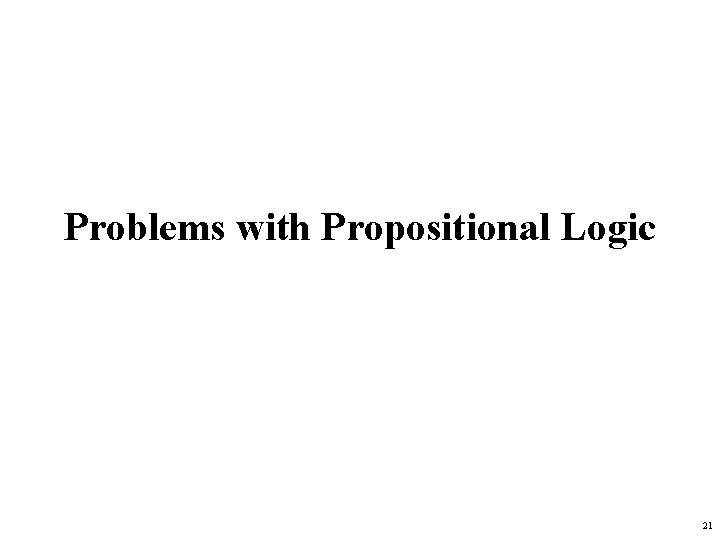Problems with Propositional Logic 21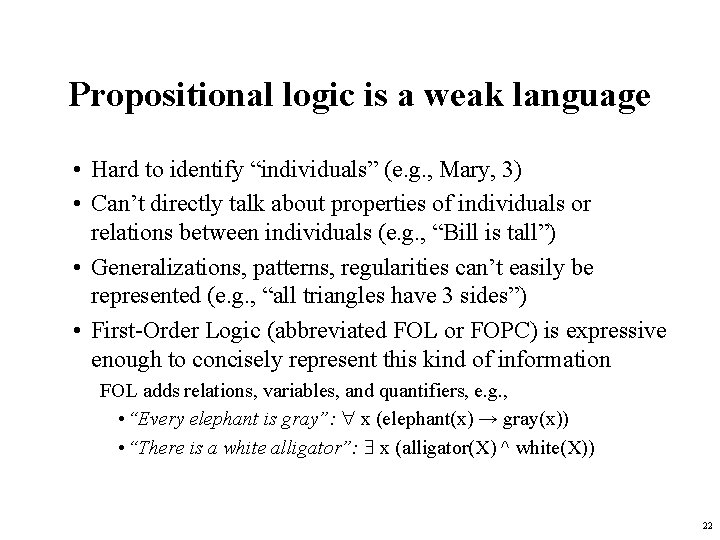Propositional logic is a weak language • Hard to identify “individuals” (e. g. , Mary, 3) • Can’t directly talk about properties of individuals or relations between individuals (e. g. , “Bill is tall”) • Generalizations, patterns, regularities can’t easily be represented (e. g. , “all triangles have 3 sides”) • First-Order Logic (abbreviated FOL or FOPC) is expressive enough to concisely represent this kind of information FOL adds relations, variables, and quantifiers, e. g. , • “Every elephant is gray”: x (elephant(x) → gray(x)) • “There is a white alligator”: x (alligator(X) ^ white(X)) 22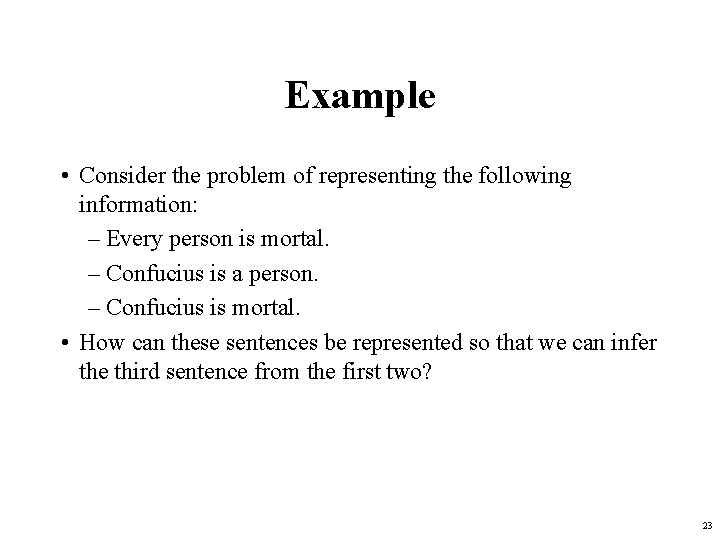Example • Consider the problem of representing the following information: – Every person is mortal. – Confucius is a person. – Confucius is mortal. • How can these sentences be represented so that we can infer the third sentence from the first two? 23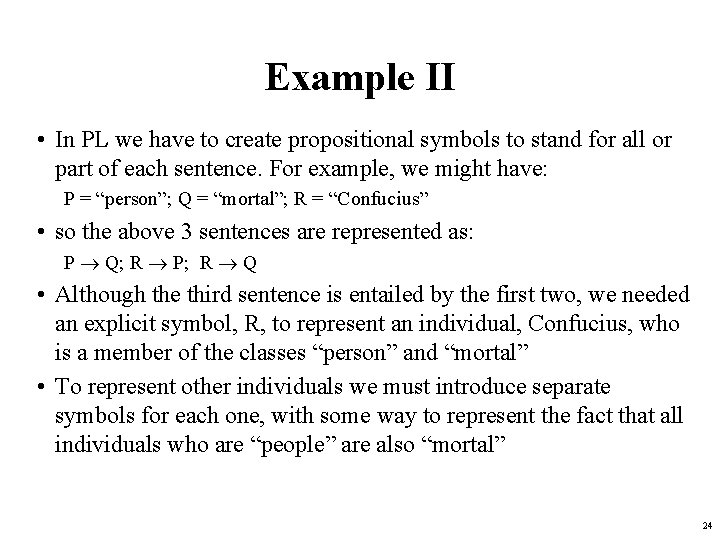Example II • In PL we have to create propositional symbols to stand for all or part of each sentence. For example, we might have: P = “person”; Q = “mortal”; R = “Confucius” • so the above 3 sentences are represented as: P Q; R P; R Q • Although the third sentence is entailed by the first two, we needed an explicit symbol, R, to represent an individual, Confucius, who is a member of the classes “person” and “mortal” • To represent other individuals we must introduce separate symbols for each one, with some way to represent the fact that all individuals who are “people” are also “mortal” 24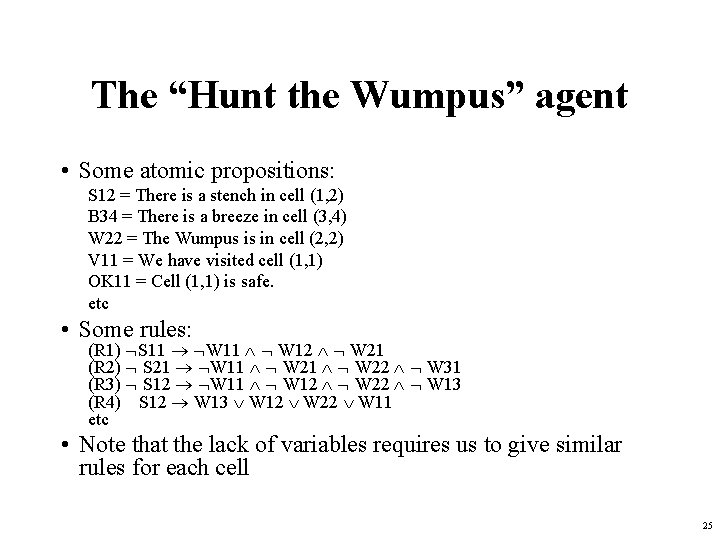The “Hunt the Wumpus” agent • Some atomic propositions: S 12 = There is a stench in cell (1, 2) B 34 = There is a breeze in cell (3, 4) W 22 = The Wumpus is in cell (2, 2) V 11 = We have visited cell (1, 1) OK 11 = Cell (1, 1) is safe. etc • Some rules: (R 1) S 11 W 11 W 12 W 21 (R 2) S 21 W 11 W 22 W 31 (R 3) S 12 W 11 W 12 W 22 W 13 (R 4) S 12 W 13 W 12 W 22 W 11 etc • Note that the lack of variables requires us to give similar rules for each cell 25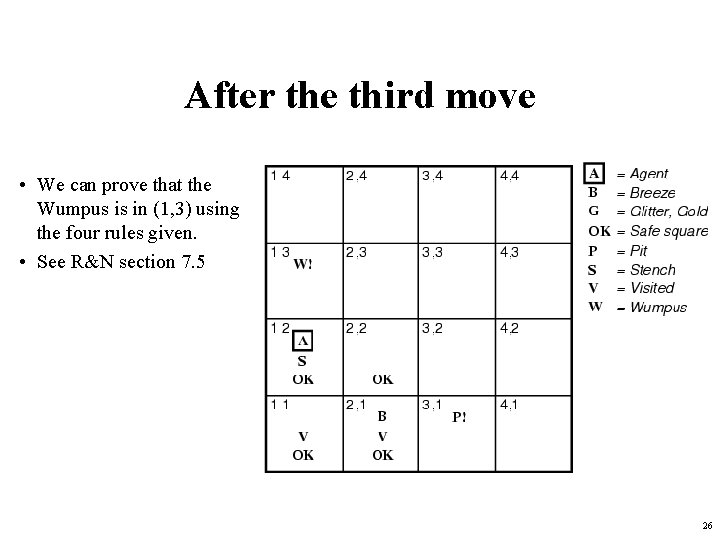After the third move • We can prove that the Wumpus is in (1, 3) using the four rules given. • See R&N section 7. 5 26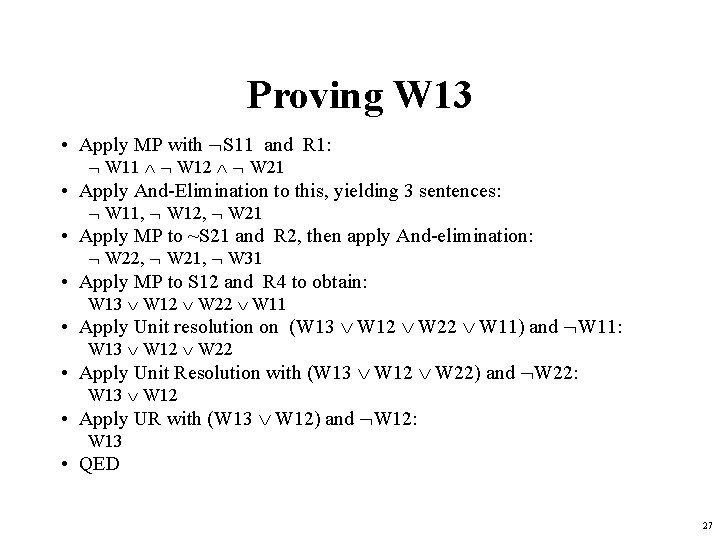Proving W 13 • Apply MP with S 11 and R 1: W 11 W 12 W 21 • Apply And-Elimination to this, yielding 3 sentences: W 11, W 12, W 21 • Apply MP to ~S 21 and R 2, then apply And-elimination: W 22, W 21, W 31 • Apply MP to S 12 and R 4 to obtain: W 13 W 12 W 22 W 11 • Apply Unit resolution on (W 13 W 12 W 22 W 11) and W 11: W 13 W 12 W 22 • Apply Unit Resolution with (W 13 W 12 W 22) and W 22: W 13 W 12 • Apply UR with (W 13 W 12) and W 12: W 13 • QED 27Problems with the propositional Wumpus hunter • Lack of variables prevents stating more general rules – We need a set of similar rules for each cell • Change of the KB over time is difficult to represent – Standard technique is to index facts with the time when they’re true – This means we have a separate KB for every time point 28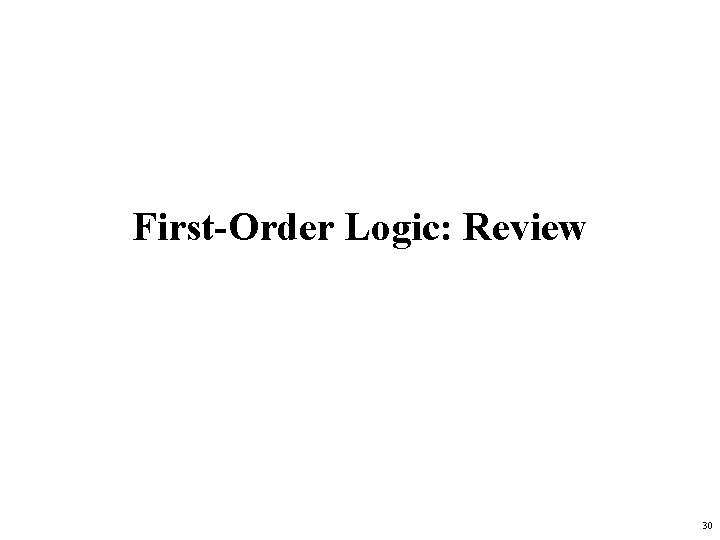First-Order Logic: Review 30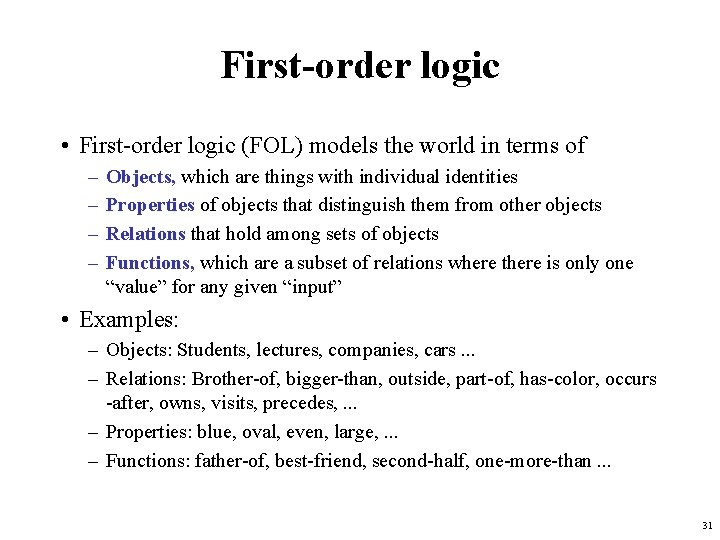First-order logic • First-order logic (FOL) models the world in terms of – – Objects, which are things with individual identities Properties of objects that distinguish them from other objects Relations that hold among sets of objects Functions, which are a subset of relations where there is only one “value” for any given “input” • Examples: – Objects: Students, lectures, companies, cars. . . – Relations: Brother-of, bigger-than, outside, part-of, has-color, occurs -after, owns, visits, precedes, . . . – Properties: blue, oval, even, large, . . . – Functions: father-of, best-friend, second-half, one-more-than. . . 31User provides • Constant symbols, which represent individuals in the world – Mary – 3 – Green • Function symbols, which map individuals to individuals – father-of(Mary) = John – color-of(Sky) = Blue • Predicate symbols, which map individuals to truth values – greater(5, 3) – green(Grass) – color(Grass, Green) 32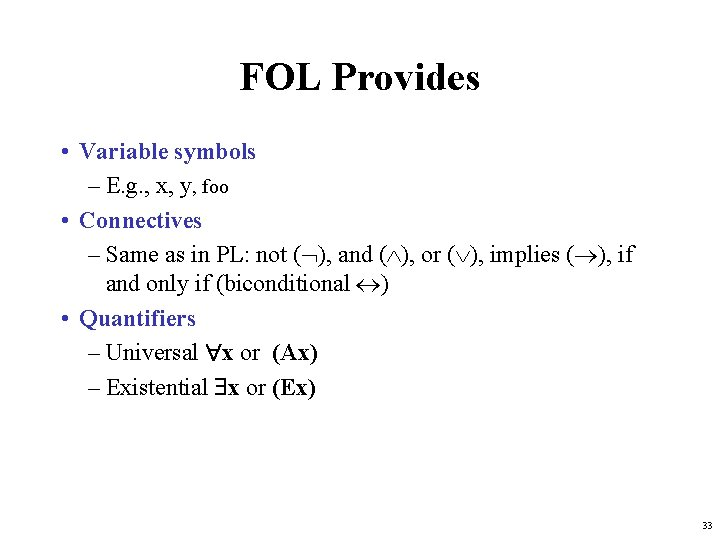FOL Provides • Variable symbols – E. g. , x, y, foo • Connectives – Same as in PL: not ( ), and ( ), or ( ), implies ( ), if and only if (biconditional ) • Quantifiers – Universal x or (Ax) – Existential x or (Ex) 33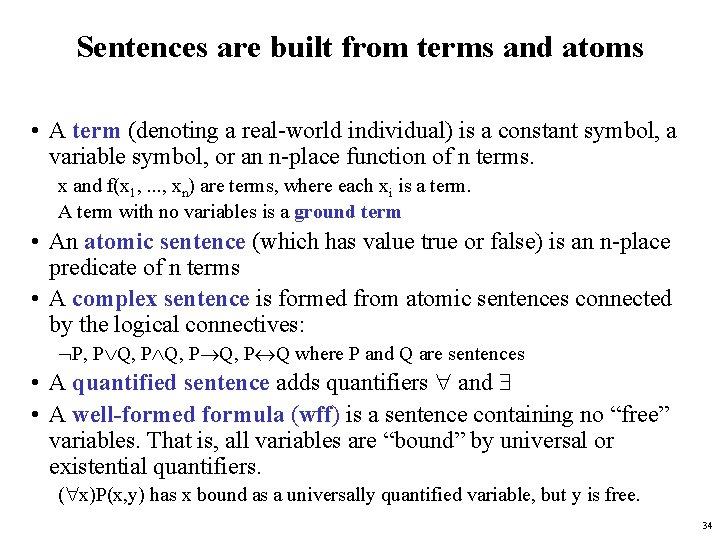Sentences are built from terms and atoms • A term (denoting a real-world individual) is a constant symbol, a variable symbol, or an n-place function of n terms. x and f(x 1, . . . , xn) are terms, where each xi is a term. A term with no variables is a ground term • An atomic sentence (which has value true or false) is an n-place predicate of n terms • A complex sentence is formed from atomic sentences connected by the logical connectives: P, P Q, P Q where P and Q are sentences • A quantified sentence adds quantifiers and • A well-formed formula (wff) is a sentence containing no “free” variables. That is, all variables are “bound” by universal or existential quantifiers. ( x)P(x, y) has x bound as a universally quantified variable, but y is free. 34Quantifiers • Universal quantification – ( x)P(x) means that P holds for all values of x in the domain associated with that variable – E. g. , ( x) dolphin(x) mammal(x) • Existential quantification – ( x)P(x) means that P holds for some value of x in the domain associated with that variable – E. g. , ( x) mammal(x) lays-eggs(x) – Permits one to make a statement about some object without naming it 36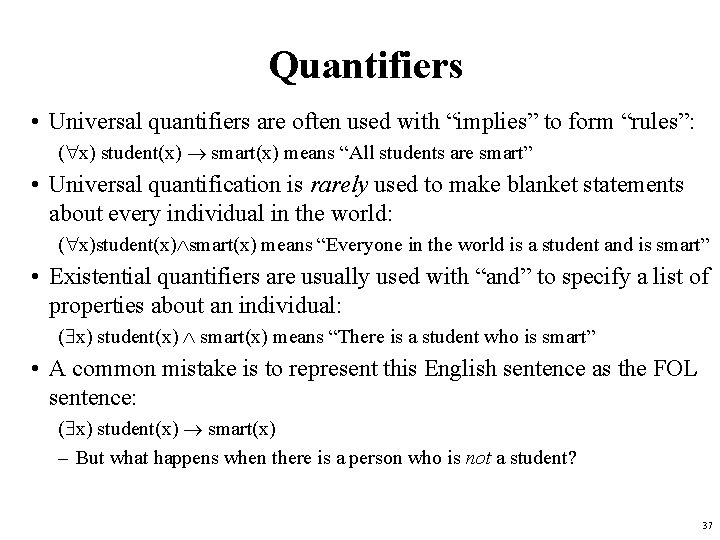Quantifiers • Universal quantifiers are often used with “implies” to form “rules”: ( x) student(x) smart(x) means “All students are smart” • Universal quantification is rarely used to make blanket statements about every individual in the world: ( x)student(x) smart(x) means “Everyone in the world is a student and is smart” • Existential quantifiers are usually used with “and” to specify a list of properties about an individual: ( x) student(x) smart(x) means “There is a student who is smart” • A common mistake is to represent this English sentence as the FOL sentence: ( x) student(x) smart(x) – But what happens when there is a person who is not a student? 37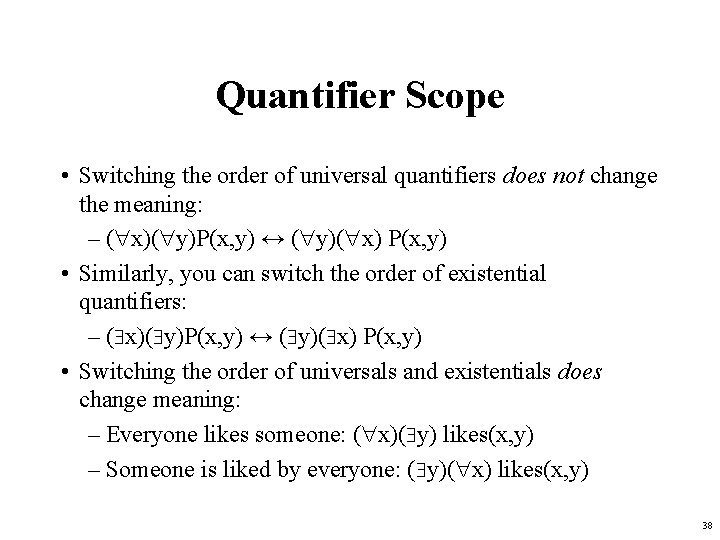Quantifier Scope • Switching the order of universal quantifiers does not change the meaning: – ( x)( y)P(x, y) ↔ ( y)( x) P(x, y) • Similarly, you can switch the order of existential quantifiers: – ( x)( y)P(x, y) ↔ ( y)( x) P(x, y) • Switching the order of universals and existentials does change meaning: – Everyone likes someone: ( x)( y) likes(x, y) – Someone is liked by everyone: ( y)( x) likes(x, y) 38Connections between All and Exists We can relate sentences involving and using De Morgan’s laws: ( x) P(x) ↔ ( x) P(x) ↔ ( x) P(x) ↔ ( x) P(x) 39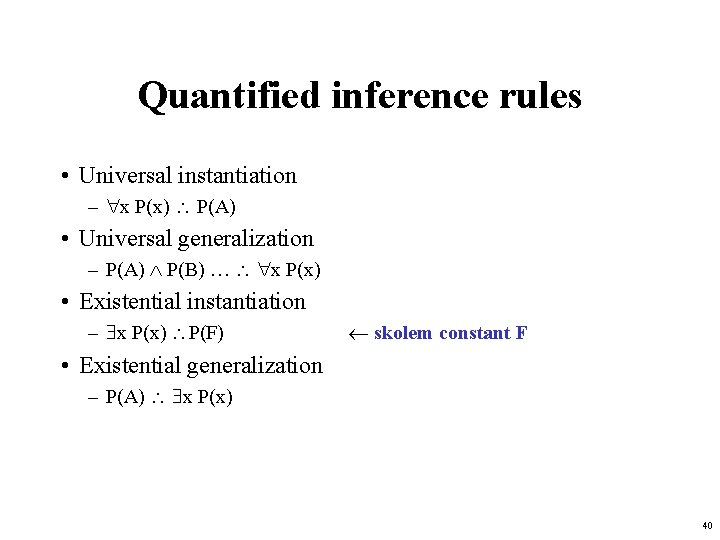Quantified inference rules • Universal instantiation – x P(x) P(A) • Universal generalization – P(A) P(B) … x P(x) • Existential instantiation – x P(x) P(F) skolem constant F • Existential generalization – P(A) x P(x) 40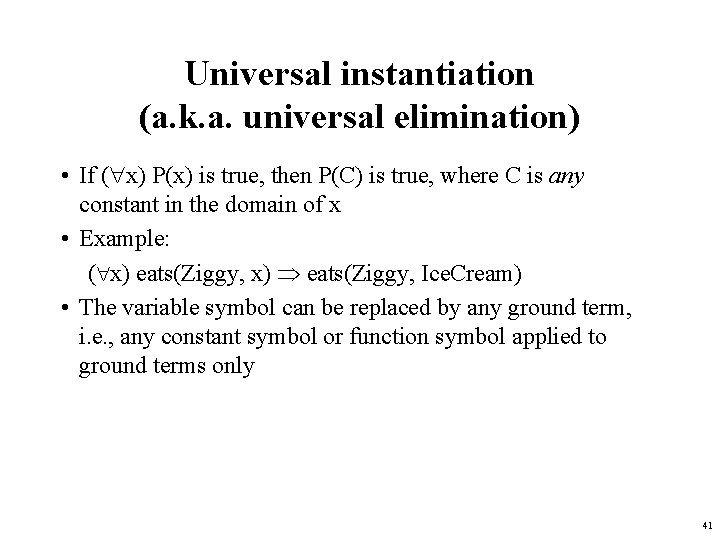Universal instantiation (a. k. a. universal elimination) • If ( x) P(x) is true, then P(C) is true, where C is any constant in the domain of x • Example: ( x) eats(Ziggy, x) eats(Ziggy, Ice. Cream) • The variable symbol can be replaced by any ground term, i. e. , any constant symbol or function symbol applied to ground terms only 41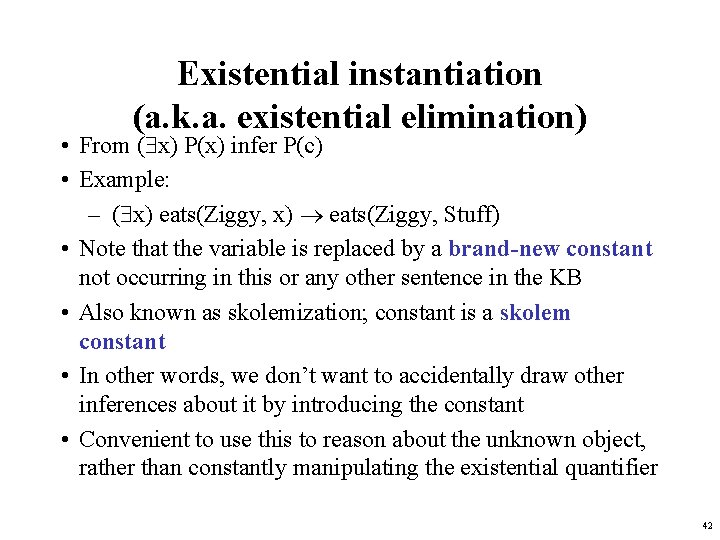Existential instantiation (a. k. a. existential elimination) • From ( x) P(x) infer P(c) • Example: – ( x) eats(Ziggy, x) eats(Ziggy, Stuff) • Note that the variable is replaced by a brand-new constant not occurring in this or any other sentence in the KB • Also known as skolemization; constant is a skolem constant • In other words, we don’t want to accidentally draw other inferences about it by introducing the constant • Convenient to use this to reason about the unknown object, rather than constantly manipulating the existential quantifier 42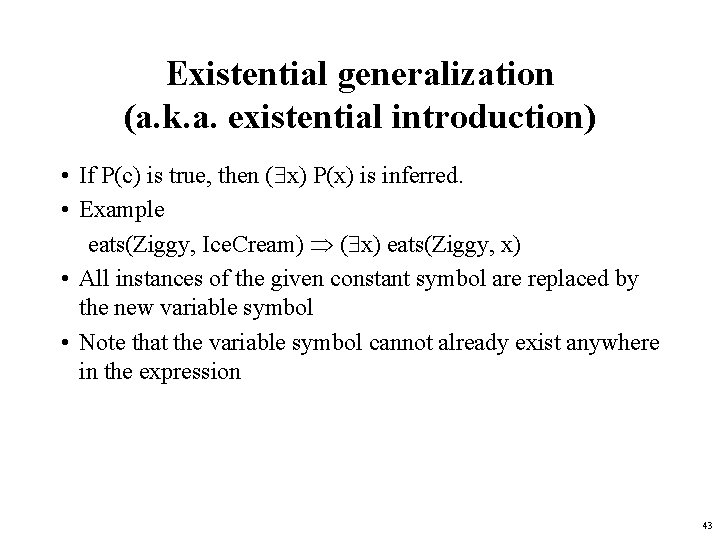Existential generalization (a. k. a. existential introduction) • If P(c) is true, then ( x) P(x) is inferred. • Example eats(Ziggy, Ice. Cream) ( x) eats(Ziggy, x) • All instances of the given constant symbol are replaced by the new variable symbol • Note that the variable symbol cannot already exist anywhere in the expression 43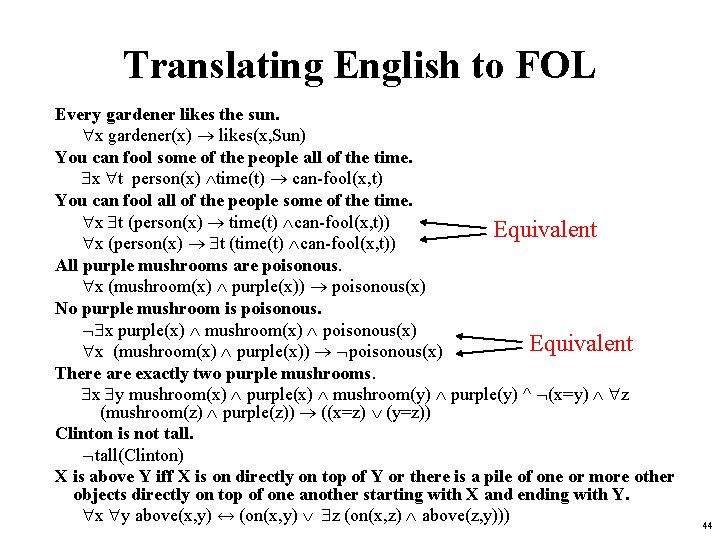Translating English to FOL Every gardener likes the sun. x gardener(x) likes(x, Sun) You can fool some of the people all of the time. x t person(x) time(t) can-fool(x, t) You can fool all of the people some of the time. x t (person(x) time(t) can-fool(x, t)) Equivalent x (person(x) t (time(t) can-fool(x, t)) All purple mushrooms are poisonous. x (mushroom(x) purple(x)) poisonous(x) No purple mushroom is poisonous. x purple(x) mushroom(x) poisonous(x) Equivalent x (mushroom(x) purple(x)) poisonous(x) There are exactly two purple mushrooms. x y mushroom(x) purple(x) mushroom(y) purple(y) ^ (x=y) z (mushroom(z) purple(z)) ((x=z) (y=z)) Clinton is not tall(Clinton) X is above Y iff X is on directly on top of Y or there is a pile of one or more other objects directly on top of one another starting with X and ending with Y. x y above(x, y) ↔ (on(x, y) z (on(x, z) above(z, y))) 44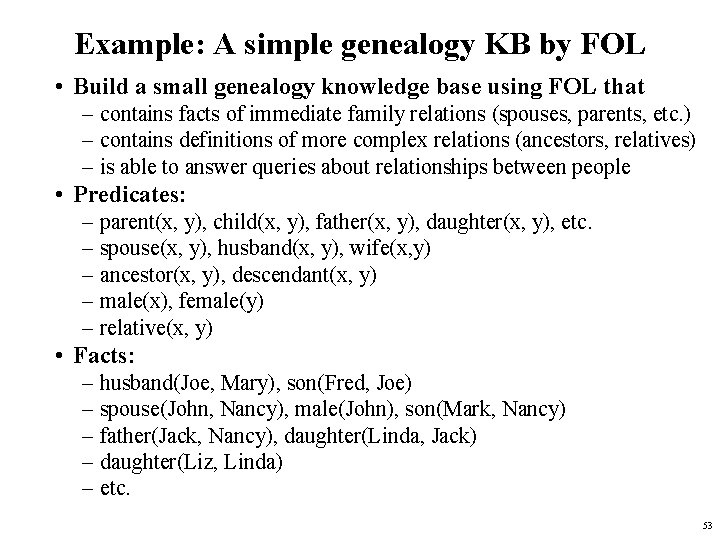Example: A simple genealogy KB by FOL • Build a small genealogy knowledge base using FOL that – contains facts of immediate family relations (spouses, parents, etc. ) – contains definitions of more complex relations (ancestors, relatives) – is able to answer queries about relationships between people • Predicates: – parent(x, y), child(x, y), father(x, y), daughter(x, y), etc. – spouse(x, y), husband(x, y), wife(x, y) – ancestor(x, y), descendant(x, y) – male(x), female(y) – relative(x, y) • Facts: – husband(Joe, Mary), son(Fred, Joe) – spouse(John, Nancy), male(John), son(Mark, Nancy) – father(Jack, Nancy), daughter(Linda, Jack) – daughter(Liz, Linda) – etc. 53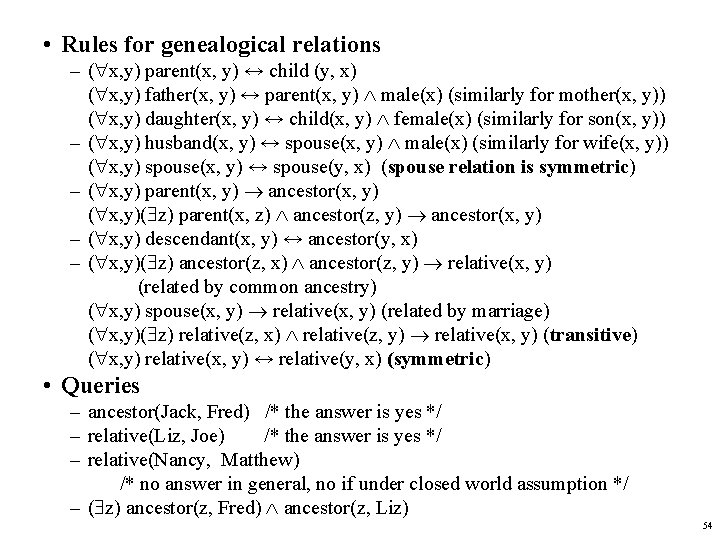• Rules for genealogical relations – ( x, y) parent(x, y) ↔ child (y, x) ( x, y) father(x, y) ↔ parent(x, y) male(x) (similarly for mother(x, y)) ( x, y) daughter(x, y) ↔ child(x, y) female(x) (similarly for son(x, y)) – ( x, y) husband(x, y) ↔ spouse(x, y) male(x) (similarly for wife(x, y)) ( x, y) spouse(x, y) ↔ spouse(y, x) (spouse relation is symmetric) – ( x, y) parent(x, y) ancestor(x, y) ( x, y)( z) parent(x, z) ancestor(z, y) ancestor(x, y) – ( x, y) descendant(x, y) ↔ ancestor(y, x) – ( x, y)( z) ancestor(z, x) ancestor(z, y) relative(x, y) (related by common ancestry) ( x, y) spouse(x, y) relative(x, y) (related by marriage) ( x, y)( z) relative(z, x) relative(z, y) relative(x, y) (transitive) ( x, y) relative(x, y) ↔ relative(y, x) (symmetric) • Queries – ancestor(Jack, Fred) /* the answer is yes */ – relative(Liz, Joe) /* the answer is yes */ – relative(Nancy, Matthew) /* no answer in general, no if under closed world assumption */ – ( z) ancestor(z, Fred) ancestor(z, Liz) 54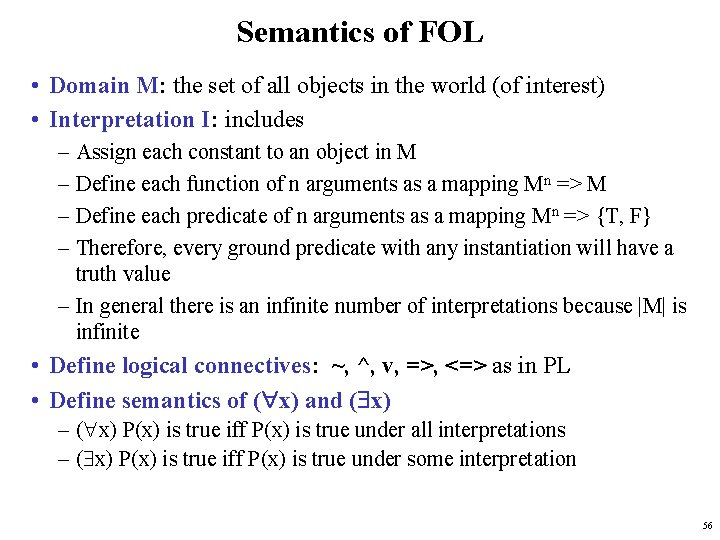Semantics of FOL • Domain M: the set of all objects in the world (of interest) • Interpretation I: includes – Assign each constant to an object in M – Define each function of n arguments as a mapping Mn => M – Define each predicate of n arguments as a mapping Mn => {T, F} – Therefore, every ground predicate with any instantiation will have a truth value – In general there is an infinite number of interpretations because |M| is infinite • Define logical connectives: ~, ^, v, =>, <=> as in PL • Define semantics of ( x) and ( x) – ( x) P(x) is true iff P(x) is true under all interpretations – ( x) P(x) is true iff P(x) is true under some interpretation 56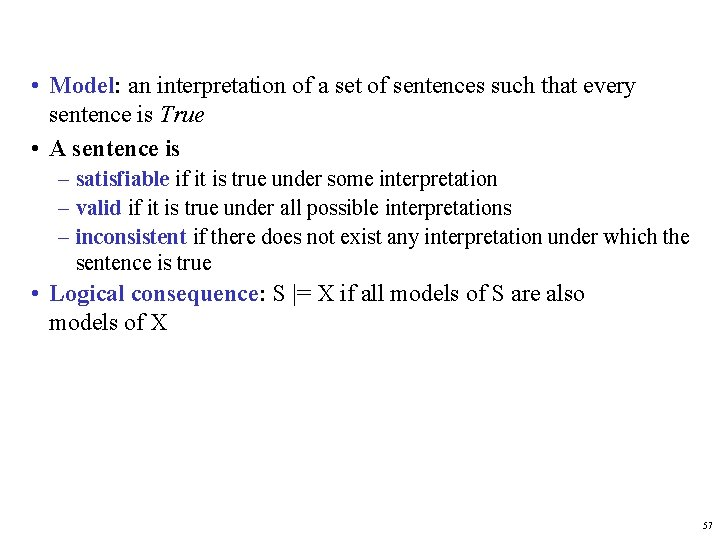• Model: an interpretation of a set of sentences such that every sentence is True • A sentence is – satisfiable if it is true under some interpretation – valid if it is true under all possible interpretations – inconsistent if there does not exist any interpretation under which the sentence is true • Logical consequence: S |= X if all models of S are also models of X 57Axioms, definitions and theorems • Axioms are facts and rules that attempt to capture all of the (important) facts and concepts about a domain; axioms can be used to prove theorems –Mathematicians don’t want any unnecessary (dependent) axioms –ones that can be derived from other axioms –Dependent axioms can make reasoning faster, however –Choosing a good set of axioms for a domain is a kind of design problem • A definition of a predicate is of the form “p(X) ↔ …” and can be decomposed into two parts –Necessary description: “p(x) …” –Sufficient description “p(x) …” –Some concepts don’t have complete definitions (e. g. , person(x)) 58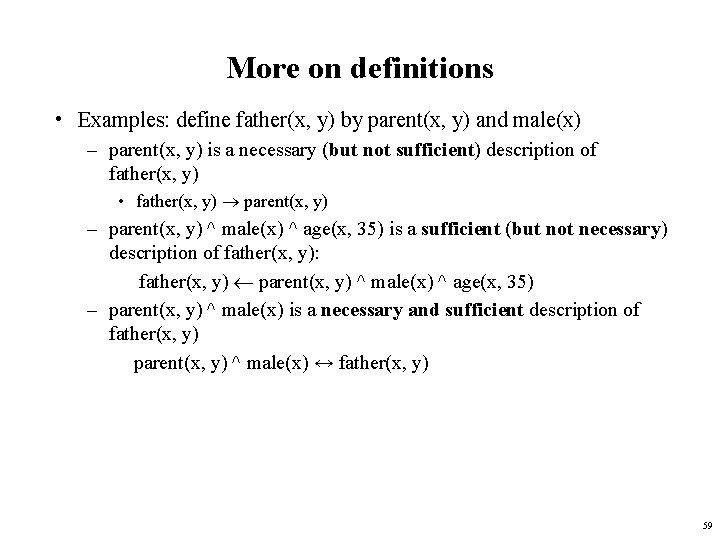More on definitions • Examples: define father(x, y) by parent(x, y) and male(x) – parent(x, y) is a necessary (but not sufficient) description of father(x, y) • father(x, y) parent(x, y) – parent(x, y) ^ male(x) ^ age(x, 35) is a sufficient (but not necessary) description of father(x, y): father(x, y) parent(x, y) ^ male(x) ^ age(x, 35) – parent(x, y) ^ male(x) is a necessary and sufficient description of father(x, y) parent(x, y) ^ male(x) ↔ father(x, y) 59More on definitions S(x) is a necessary condition of P(x) S(x) is a sufficient condition of P(x) S(x) is a necessary and sufficient condition of P(x) S(x) ( x) P(x) => S(x) ( x) P(x) <=> S(x) 60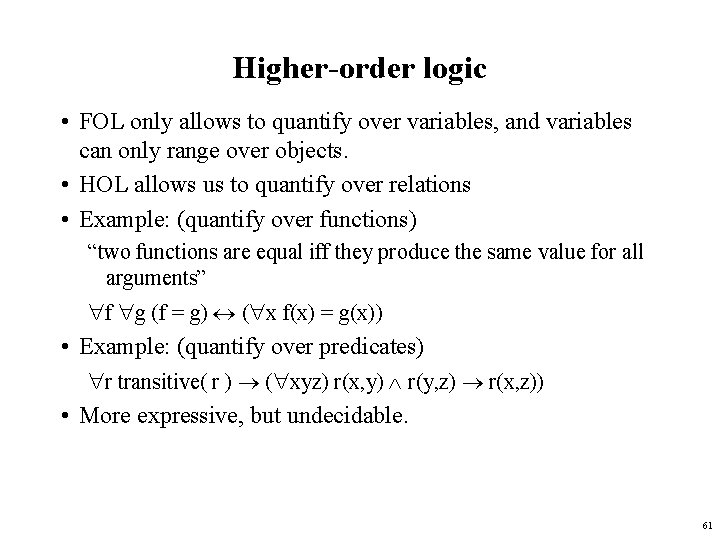Higher-order logic • FOL only allows to quantify over variables, and variables can only range over objects. • HOL allows us to quantify over relations • Example: (quantify over functions) “two functions are equal iff they produce the same value for all arguments” f g (f = g) ( x f(x) = g(x)) • Example: (quantify over predicates) r transitive( r ) ( xyz) r(x, y) r(y, z) r(x, z)) • More expressive, but undecidable. 61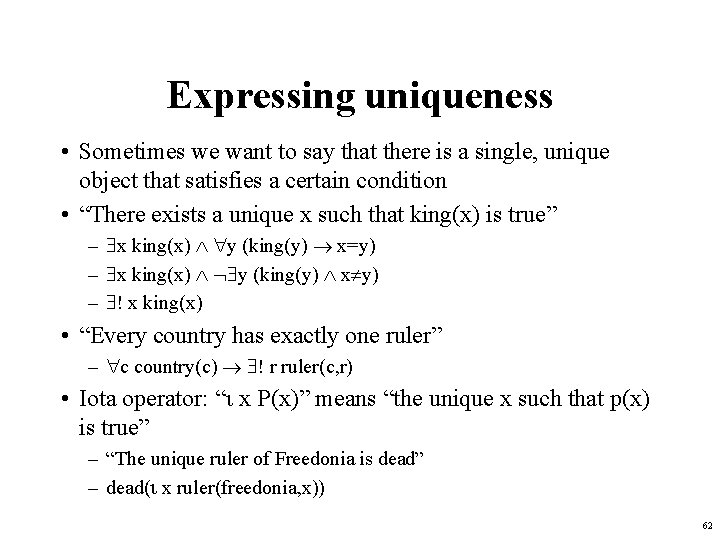Expressing uniqueness • Sometimes we want to say that there is a single, unique object that satisfies a certain condition • “There exists a unique x such that king(x) is true” – x king(x) y (king(y) x=y) – x king(x) y (king(y) x y) – ! x king(x) • “Every country has exactly one ruler” – c country(c) ! r ruler(c, r) • Iota operator: “ x P(x)” means “the unique x such that p(x) is true” – “The unique ruler of Freedonia is dead” – dead( x ruler(freedonia, x)) 62Notational differences • Different symbols for and, or, not, implies, . . . – – p v (q ^ r) p + (q * r) etc • Prolog cat(X) : - furry(X), meows (X), has(X, claws) • Lispy notations (forall ? x (implies (and (furry ? x) (meows ? x) (has ? x claws)) (cat ? x))) 63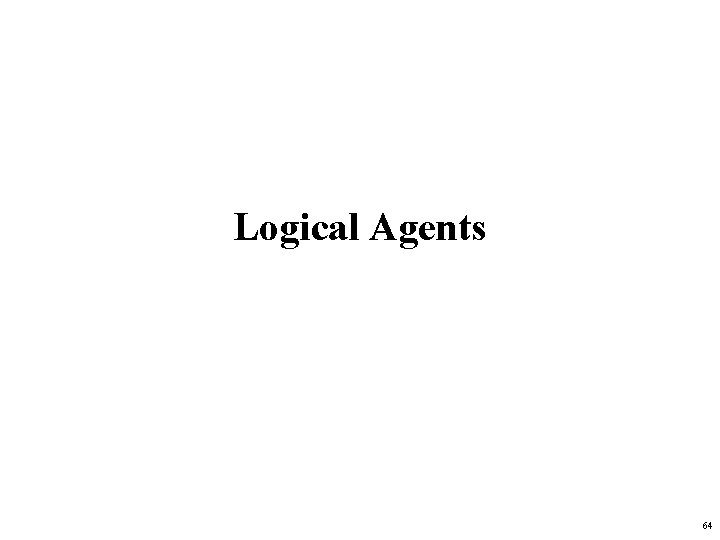Logical Agents 64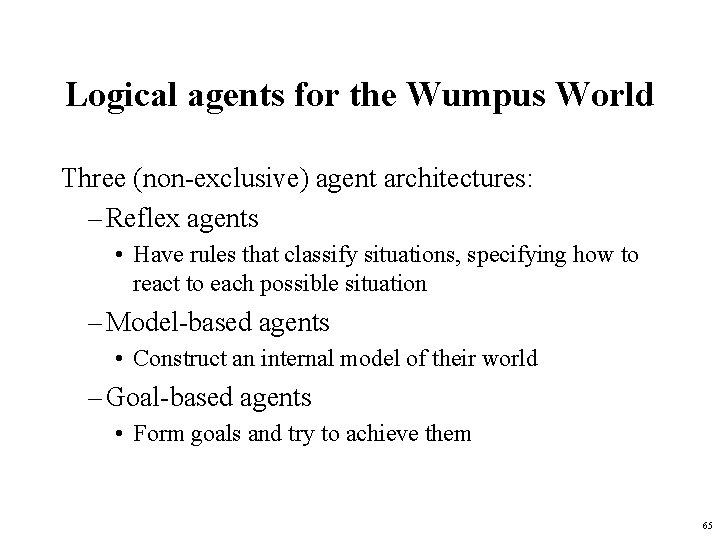Logical agents for the Wumpus World Three (non-exclusive) agent architectures: – Reflex agents • Have rules that classify situations, specifying how to react to each possible situation – Model-based agents • Construct an internal model of their world – Goal-based agents • Form goals and try to achieve them 65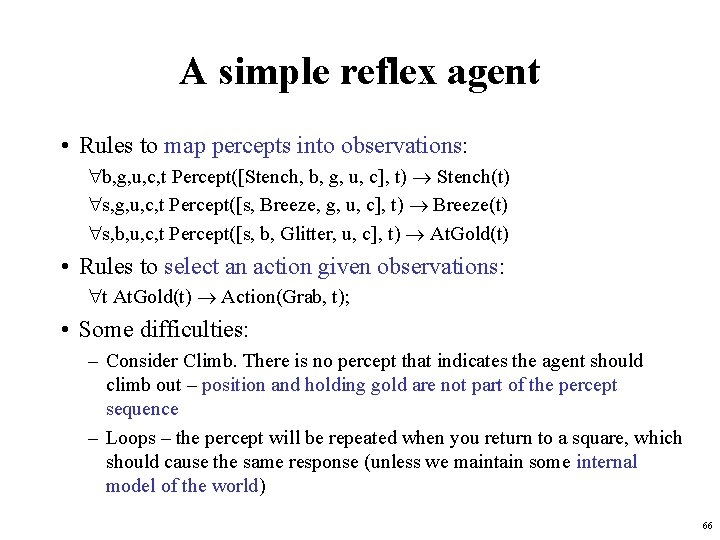A simple reflex agent • Rules to map percepts into observations: b, g, u, c, t Percept([Stench, b, g, u, c], t) Stench(t) s, g, u, c, t Percept([s, Breeze, g, u, c], t) Breeze(t) s, b, u, c, t Percept([s, b, Glitter, u, c], t) At. Gold(t) • Rules to select an action given observations: t At. Gold(t) Action(Grab, t); • Some difficulties: – Consider Climb. There is no percept that indicates the agent should climb out – position and holding gold are not part of the percept sequence – Loops – the percept will be repeated when you return to a square, which should cause the same response (unless we maintain some internal model of the world) 66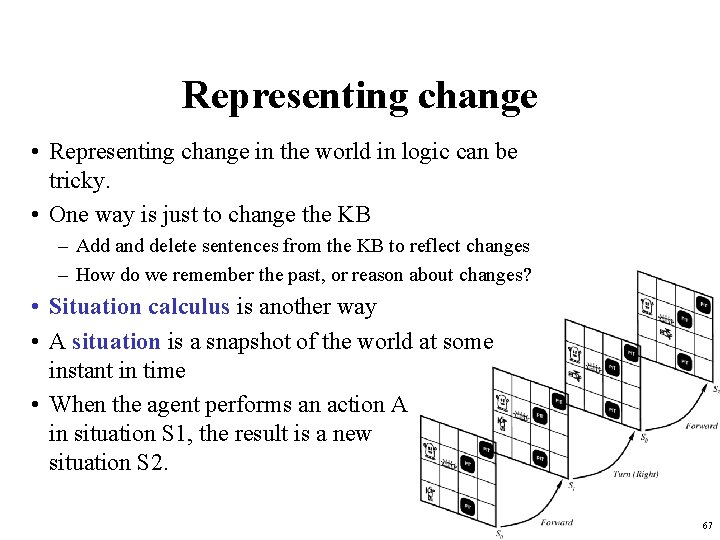Representing change • Representing change in the world in logic can be tricky. • One way is just to change the KB – Add and delete sentences from the KB to reflect changes – How do we remember the past, or reason about changes? • Situation calculus is another way • A situation is a snapshot of the world at some instant in time • When the agent performs an action A in situation S 1, the result is a new situation S 2. 67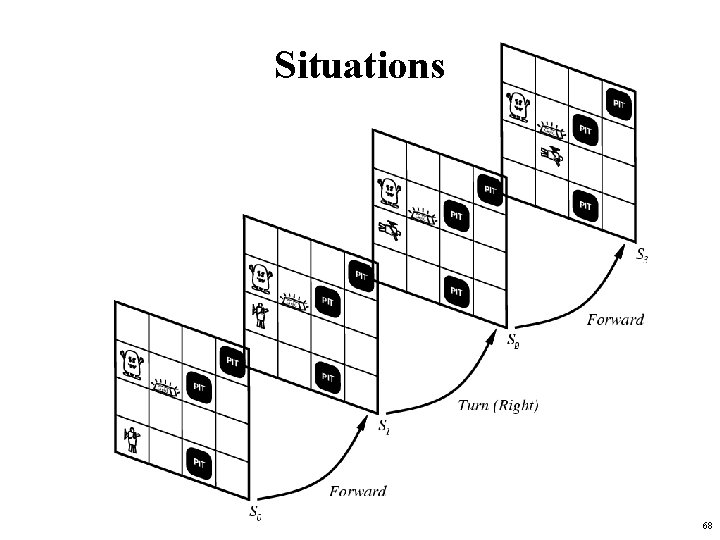Situations 68Situation calculus • A situation is a snapshot of the world at an interval of time during which nothing changes • Every true or false statement is made with respect to a particular situation. – Add situation variables to every predicate. – at(Agent, 1, 1) becomes at(Agent, 1, 1, s 0): at(Agent, 1, 1) is true in situation (i. e. , state) s 0. – Alernatively, add a special 2 nd-order predicate, holds(f, s), that means “f is true in situation s. ” E. g. , holds(at(Agent, 1, 1), s 0) • Add a new function, result(a, s), that maps a situation s into a new situation as a result of performing action a. For example, result(forward, s) is a function that returns the successor state (situation) to s • Example: The action agent-walks-to-location-y could be represented by – ( x)( y)( s) (at(Agent, x, s) onbox(s)) at(Agent, y, result(walk(y), s)) 69Deducing hidden properties • From the perceptual information we obtain in situations, we can infer properties of locations l, s at(Agent, l, s) Breeze(s) Breezy(l) l, s at(Agent, l, s) Stench(s) Smelly(l) • Neither Breezy nor Smelly need situation arguments because pits and Wumpuses do not move around 70Deducing hidden properties II • We need to write some rules that relate various aspects of a single world state (as opposed to across states) • There are two main kinds of such rules: – Causal rules reflect the assumed direction of causality in the world: ( l 1, l 2, s) At(Wumpus, l 1, s) Adjacent(l 1, l 2) Smelly(l 2) ( l 1, l 2, s) At(Pit, l 1, s) Adjacent(l 1, l 2) Breezy(l 2) Systems that reason with causal rules are called model-based reasoning systems – Diagnostic rules infer the presence of hidden properties directly from the percept-derived information. We have already seen two diagnostic rules: ( l, s) At(Agent, l, s) Breeze(s) Breezy(l) ( l, s) At(Agent, l, s) Stench(s) Smelly(l) 71Representing change: The frame problem • Frame axioms: If property x doesn’t change as a result of applying action a in state s, then it stays the same. – On (x, z, s) Clear (x, s) On (x, table, Result(Move(x, table), s)) On(x, z, Result (Move (x, table), s)) – On (y, z, s) y x On (y, z, Result (Move (x, table), s)) – The proliferation of frame axioms becomes very cumbersome in complex domains 72The frame problem II • Successor-state axiom: General statement that characterizes every way in which a particular predicate can become true: – Either it can be made true, or it can already be true and not be changed: – On (x, table, Result(a, s)) [On (x, z, s) Clear (x, s) a = Move(x, table)] [On (x, table, s) a Move (x, z)] • In complex worlds, where you want to reason about longer chains of action, even these types of axioms are too cumbersome – Planning systems use special-purpose inference methods to reason about the expected state of the world at any point in time during a multi-step plan 73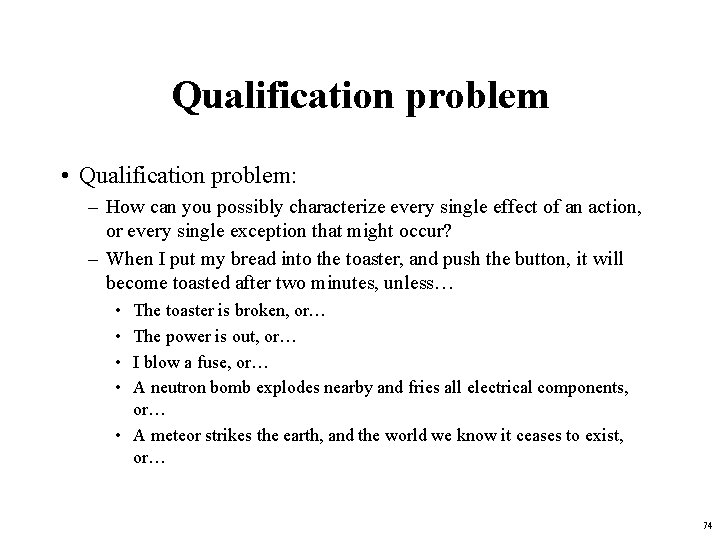Qualification problem • Qualification problem: – How can you possibly characterize every single effect of an action, or every single exception that might occur? – When I put my bread into the toaster, and push the button, it will become toasted after two minutes, unless… • • The toaster is broken, or… The power is out, or… I blow a fuse, or… A neutron bomb explodes nearby and fries all electrical components, or… • A meteor strikes the earth, and the world we know it ceases to exist, or… 74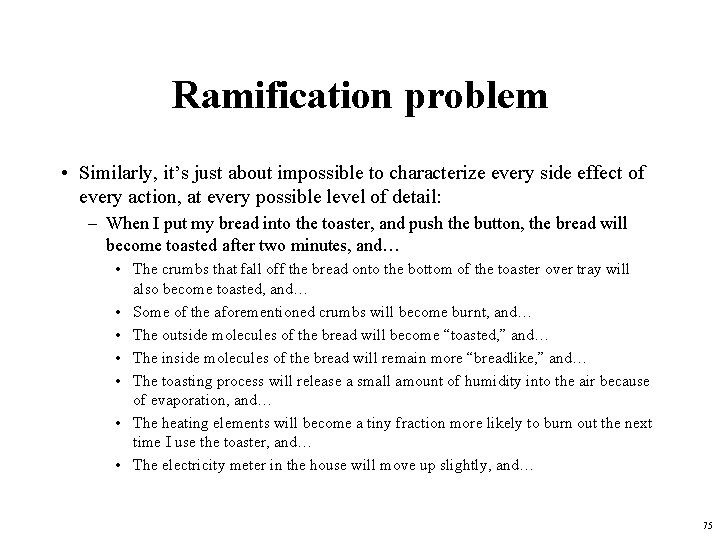Ramification problem • Similarly, it’s just about impossible to characterize every side effect of every action, at every possible level of detail: – When I put my bread into the toaster, and push the button, the bread will become toasted after two minutes, and… • The crumbs that fall off the bread onto the bottom of the toaster over tray will also become toasted, and… • Some of the aforementioned crumbs will become burnt, and… • The outside molecules of the bread will become “toasted, ” and… • The inside molecules of the bread will remain more “breadlike, ” and… • The toasting process will release a small amount of humidity into the air because of evaporation, and… • The heating elements will become a tiny fraction more likely to burn out the next time I use the toaster, and… • The electricity meter in the house will move up slightly, and… 75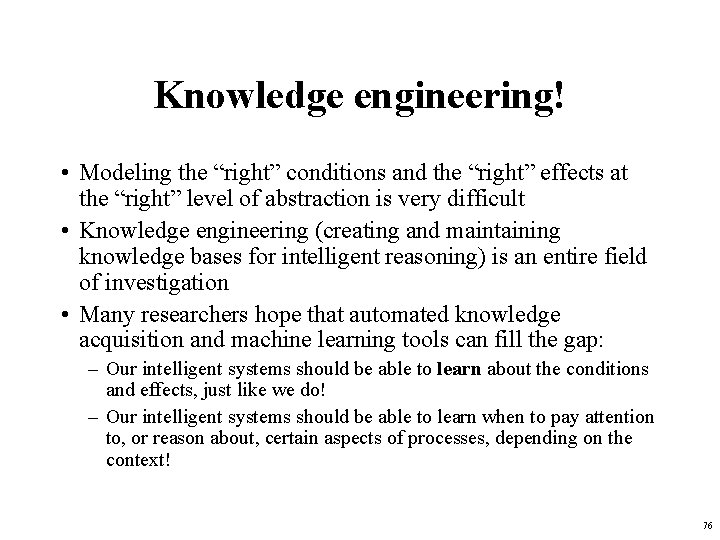Knowledge engineering! • Modeling the “right” conditions and the “right” effects at the “right” level of abstraction is very difficult • Knowledge engineering (creating and maintaining knowledge bases for intelligent reasoning) is an entire field of investigation • Many researchers hope that automated knowledge acquisition and machine learning tools can fill the gap: – Our intelligent systems should be able to learn about the conditions and effects, just like we do! – Our intelligent systems should be able to learn when to pay attention to, or reason about, certain aspects of processes, depending on the context! 76Preferences among actions • A problem with the Wumpus world knowledge base that we have built so far is that it is difficult to decide which action is best among a number of possibilities. • For example, to decide between a forward and a grab, axioms describing when it is OK to move to a square would have to mention glitter. • This is not modular! • We can solve this problem by separating facts about actions from facts about goals. This way our agent can be reprogrammed just by asking it to achieve different goals. 77Preferences among actions • The first step is to describe the desirability of actions independent of each other. • In doing this we will use a simple scale: actions can be Great, Good, Medium, Risky, or Deadly. • Obviously, the agent should always do the best action it can find: ( a, s) Great(a, s) Action(a, s) ( a, s) Good(a, s) ( b) Great(b, s) Action(a, s) ( a, s) Medium(a, s) ( ( b) Great(b, s) Good(b, s)) Action(a, s). . . 78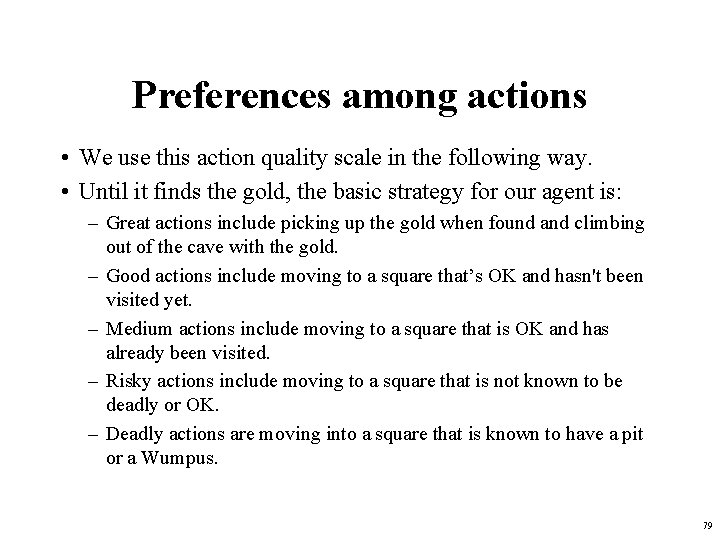Preferences among actions • We use this action quality scale in the following way. • Until it finds the gold, the basic strategy for our agent is: – Great actions include picking up the gold when found and climbing out of the cave with the gold. – Good actions include moving to a square that’s OK and hasn't been visited yet. – Medium actions include moving to a square that is OK and has already been visited. – Risky actions include moving to a square that is not known to be deadly or OK. – Deadly actions are moving into a square that is known to have a pit or a Wumpus. 79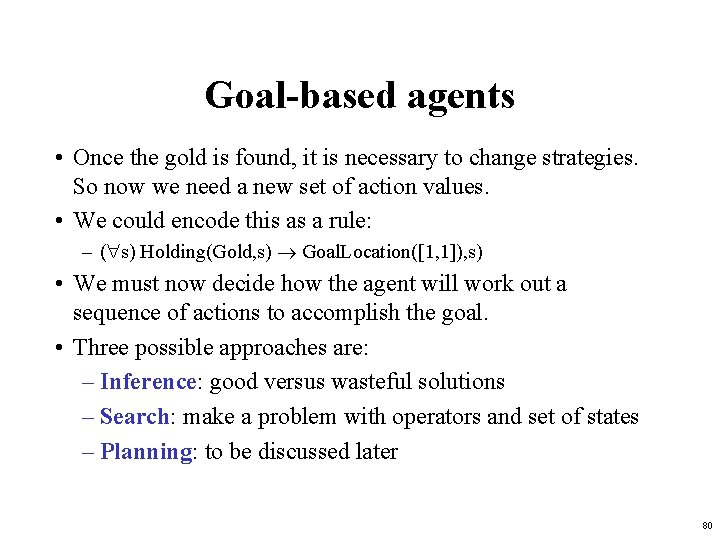Goal-based agents • Once the gold is found, it is necessary to change strategies. So now we need a new set of action values. • We could encode this as a rule: – ( s) Holding(Gold, s) Goal. Location([1, 1]), s) • We must now decide how the agent will work out a sequence of actions to accomplish the goal. • Three possible approaches are: – Inference: good versus wasteful solutions – Search: make a problem with operators and set of states – Planning: to be discussed later 80Coming up next: • Logical inference (Thursday) • Knowledge representation • Planning 81